# Thread: Solving Differential Equations with An Integrating Factor

1. ## Solving Differential Equations with An Integrating Factor

I have two differential equations that I need to solve. All the teacher told me is that I need to use a integrating factor to solve them (although I am not entirely sure he even knows what that is). So any help or links to any good resource on the subject would be great:

1. $\displaystyle \frac{dy}{dx}=2x(1+x^2-y)$

2. $\displaystyle \frac{dy}{dx}=\frac{y}{x}+\frac{y^2}{x^2}$

2.Originally Posted by flybynightI have two differential equations that I need to solve. All the teacher told me is that I need to use a integrating factor to solve them (although I am not entirely sure he even knows what that is). So any help or links to any good resource on the subject would be great:

1. $\displaystyle \frac{dy}{dx}=2x(1+x^2-y)$

2. $\displaystyle \frac{dy}{dx}=\frac{y}{x}+\frac{y^2}{x^2}$
1. Rewrite:
$\displaystyle y'+2xy=2x+2x^3$

$\displaystyle P(x)=2x$, $\displaystyle Q(x)=2x+2x^3$, and $\displaystyle e^{\int 2xdx}=e^{e^2}$

$\displaystyle \int \frac{dy}{dx}[e^{x^2}y]=\int e^{x^2}q(x)dx$

3. 2. $\displaystyle \ \ \ \frac{dy}{dx}=\frac{y}{x}+\frac{y^2}{x^2}$

$\displaystyle \Rightarrow\ \frac{dy}{dx} - \frac{y}{x}\ =\ \frac{y^2}{x^2}$

$\displaystyle \Rightarrow\ \frac{1}{y^2}\ \frac{dy}{dx} - \frac{1}{xy}\ =\ \frac{1}{x^2}$

Now, we can write this along the bottom row of...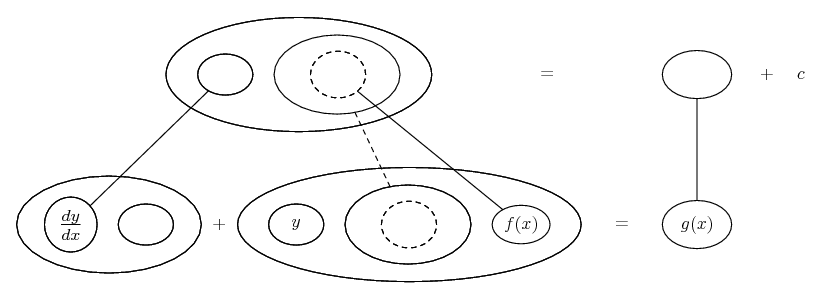... except instead of just y (as in question 1) we have a function f(y), thus...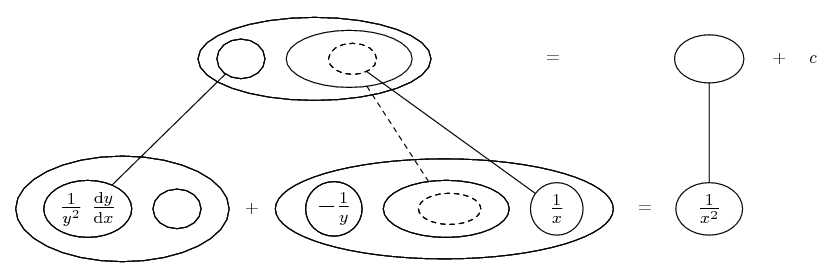... where the network on the LHS is basically the legs-uncrossed version of...... the product rule, straight lines differentiating downwards with respect to x. You use an integrating factor when you can fill out the whole of the bottom row so that the LHS is an exact product-rule derivative. To do this you generally (and in question 1 would proabably need to) take account of...... the chain rule. Straight continuous lines differentiate downwards (integrate up) with respect to x, and the straight dashed line similarly but with respect to the dashed balloon expression (which is the inner function of the composite and hence subject to the chain rule). Then you can reason thusly: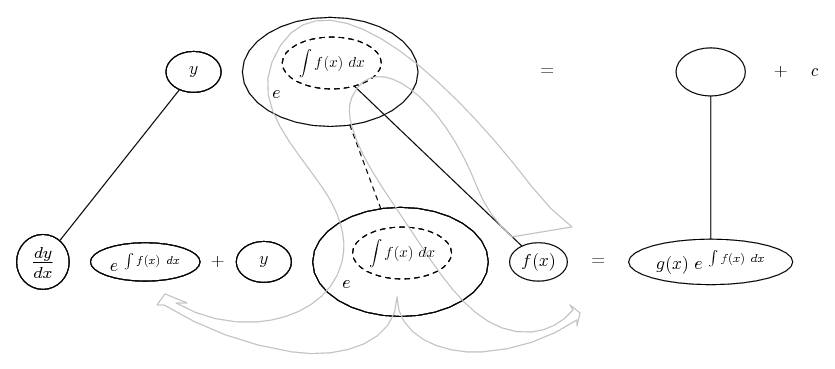(Again, this is a template in which I haven't allowed for the complication that our y term might be a function of y... but, it's still going to work.)

So in general the 'integrating factor' is e to the power of the integral of some special term in the equation that we recognise as suitable for the purpose. Then we multiply through by this exponential. In this case, though, that term being one over x, we get e to the log x, or just x.So, knowing that, we may as well zoom out of the chain rule...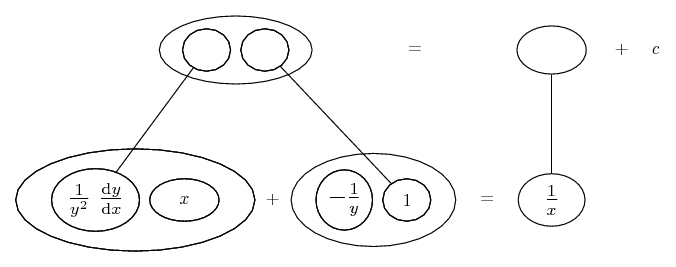We can now integrate both sides with respect to x, because the LHS is an exact product-rule derivative and the RHS is straight-forward.

However, we might want to zoom in on the chain rule to see why the complication besetting y isn't going to spoil things.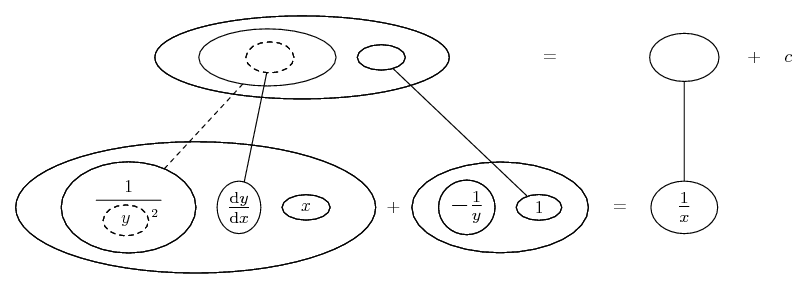Spoiler: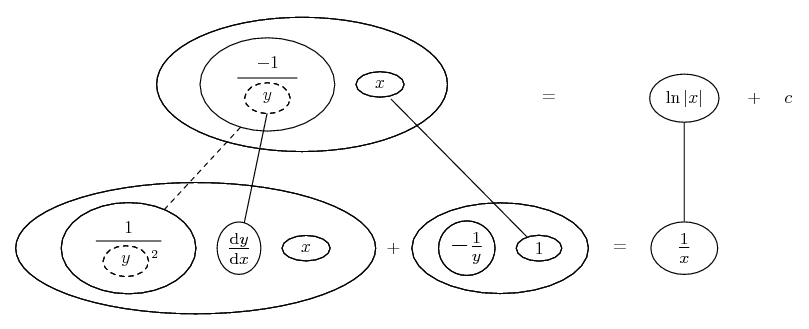i.e...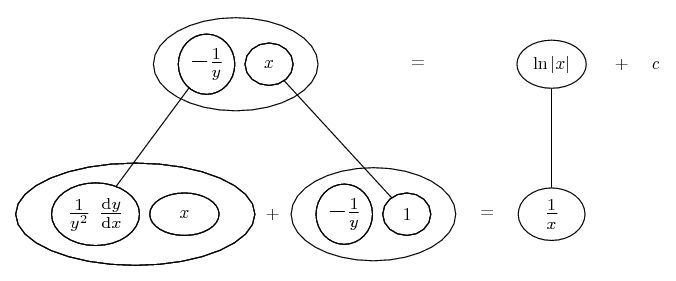The top line is your general solution, of course.

Just in case a picture helps with q.1 ...

$\displaystyle \frac{dy}{dx}=2x(1+x^2-y)$

$\displaystyle \Rightarrow\ \frac{dy}{dx} + 2xy\ =\ 2x+2x^3$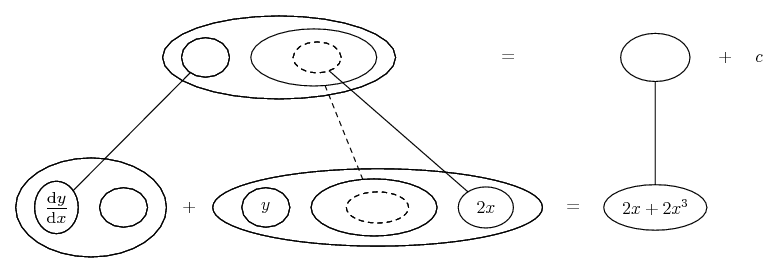Spoiler: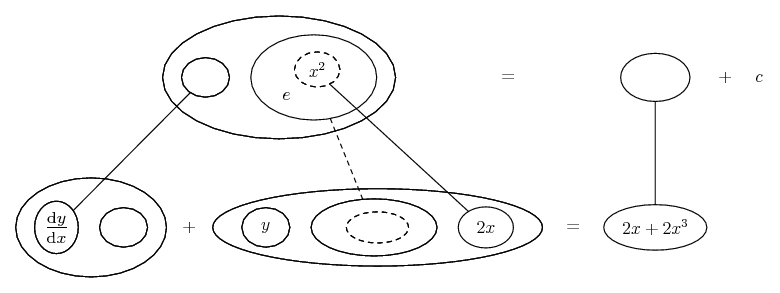Spoiler: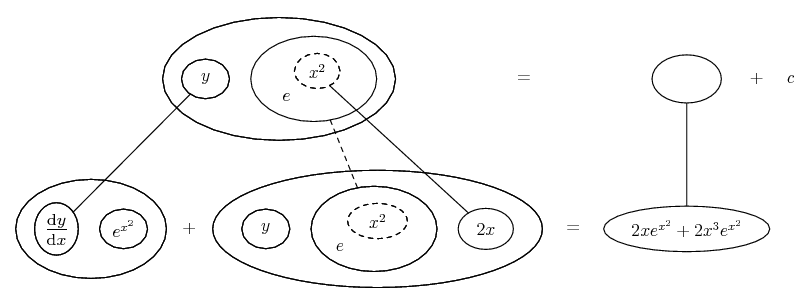For $\displaystyle 2x e^{x^2}$ we can 'spot the derivative' and use the chain rule, and for the other product we can do integration by parts, where the general drift is...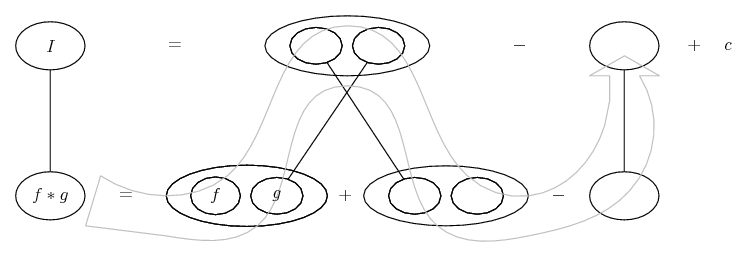Spoiler: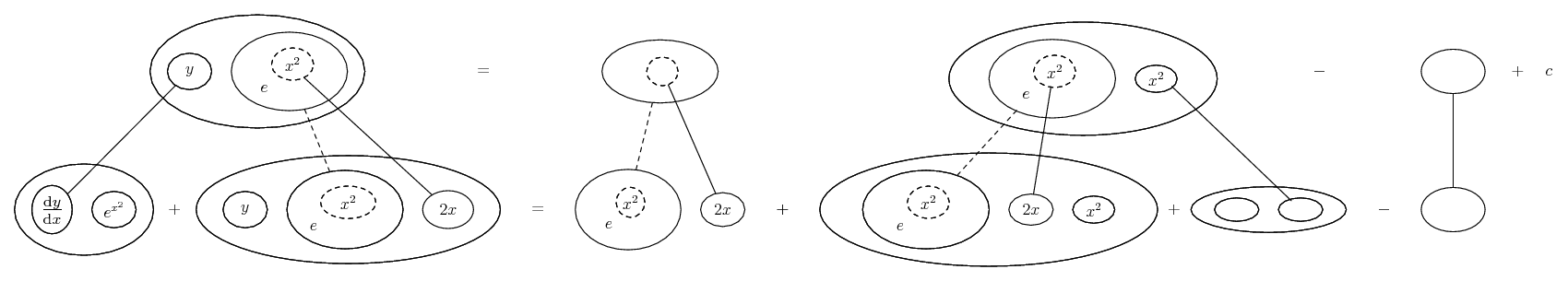However, this becomes...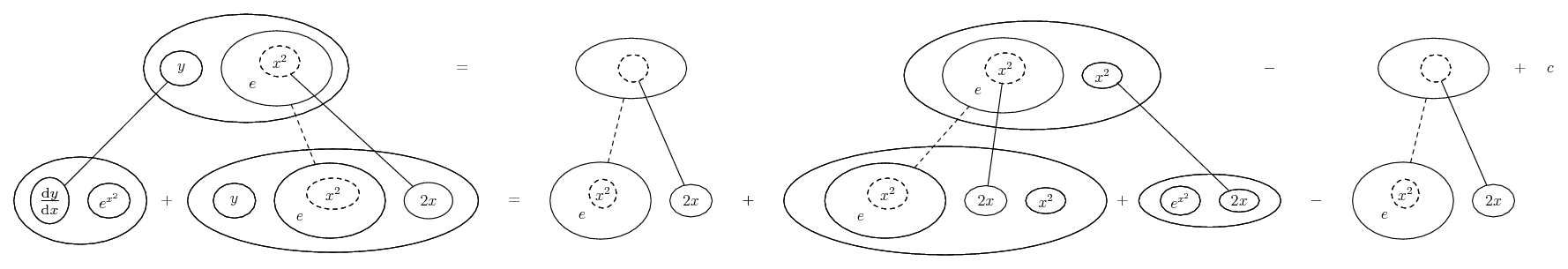So the two chain-rule shapes cancel, and what we really had all along on the RHS was another EXACT product-rule derivative!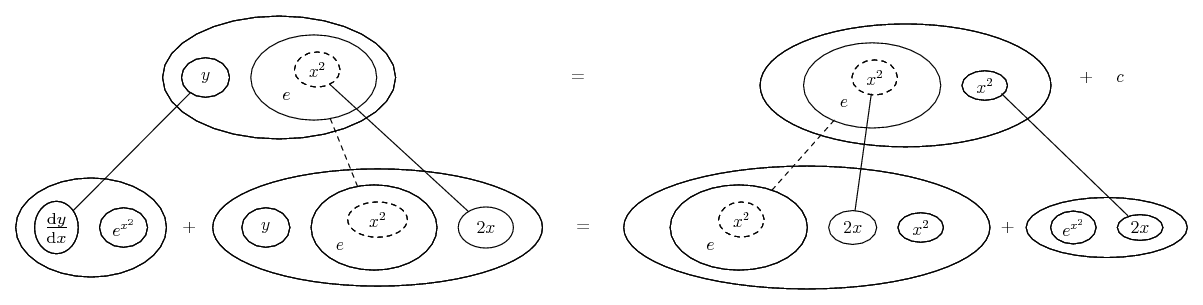(The general solution in the top row.)

__________________________________________

Don't integrate - balloontegrate!

Balloon Calculus; standard integrals, derivatives and methods

Balloon Calculus Drawing with LaTeX and Asymptote!

#### Search Tags

differential, equations, factor, integrating, solving